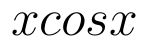Select Page

# 12 Science Maths CBSE Inverse Trigonometric Functions Answers for MCQ in English

12 Science Maths CBSE Inverse Trigonometric Functions Answers for MCQ in English to enable students to get Answers in a narrative video format for the specific question.

Expert Teacher provides 12 Science Maths CBSE Inverse Trigonometric Functions Answers for MCQ through Video Answers in English language. This video solution will be useful for students to understand how to write an answer in exam in order to score more marks. This teacher uses a narrative style for a question from Inverse Trigonometric Functions not only to explain the proper method of answering question, but deriving right answer too.

Please find the question below and view the Answer in a narrative video format.

Question:

## Similar Questions from CBSE, 12th Science, Maths, Inverse Trigonometric Functions

Question 1 : Solve the equation for(View Answer Video)

Question 2 : Write the principal value of(View Answer Video)

Question 3 : Write the principal value of(View Answer Video)

Question 4 : Write in the simplest form:(View Answer Video)

Question 5 : Ifthen value of y lies between.....? (View Answer Video)

### Three Dimensional Geometry

Question 1 : Find the vector equation of the plane with intercepts 3, -4 and 2 on x, y and z-axis respectively. (View Answer Video)

Question 2 : Write the distance between the parallel planes 2x - y + 3z = 4 and 2x - y + 3z = 18. (View Answer Video)

Question 3 : Write the vector equation of the plane passing through the point (a, b, c) and parallel to the plane(View Answer Video)

Question 4 : Find the distance of the point (-1, -5, -10) from the point of intersection of the lineand the plane(View Answer Video)

Question 5 : If linesandintersect, then find the value of k and hence find the equation of plane containing these lines. (View Answer Video)

### Application of Derivatives

Question 1 : For all real values of x the minimum value of.(View Answer Video)

Question 2 : Find two positive numbers x  and y such that x+y=60 and ismaximum. (View Answer Video)

Question 3 : The slope of the normal to the curveat x = 0 is :

Question 4 : The maximum value ofis (View Answer Video)

Question 5 : The length x of a rectangle is decreasing at the rate of 5cm/minute. and width y is increasing at the rate of 4cm/minute. When x=8cm and y=6 cm, find the rate of changes of:
Question 1 :  Find the second order derivative of the function. (View Answer Video)
Question 2 : Differentiate the functionwith respect to x. (View Answer Video)
Question 3 :  Find the second order derivative of the function. (View Answer Video)
Question 4 : Differentiate w.r.t.x the function, for some fixed a > 0 and x > 0. (View Answer Video)
Question 5 : If, findin terms of y alone. (View Answer Video)